# SSC solutions for maths Coordinate Geometry

Mathematics solutions for Coordinate Geometry class 10 SSC. Exercise 7.1, exercise 7.2, exercise 7.3, and exercise 7.4 solutions are given.

Thsese solutions are very easy to understand. First study the text book very well. Observe the example problems and solutions.

Observe the solutions and try them in your own method.

You can also see the solutions for

2. Sets

7. Coordinate geometry

10. Mensuration

11. Trigonometry

13. Probability

14. Statistics

Some solutions for different classes

Inter 1a exercise 6(a) solutions

Inter maths 1a exercise 6(b) solutions

Ncert maths class 8 exponents and powers

Ncert maths class 7 fractions and decimals

Ncert maths class 6 playing with numbers

Ncert solutions for maths class 7 rational numbers

Ncert solutions for maths class 6 knowing our numbers

Ncert solutions for maths class 6 integers

Ncert solutions for maths class 7 integers

# Coordinate Geometry solutions class 10 SSC

Exercise 7.1 Coordinate Geometry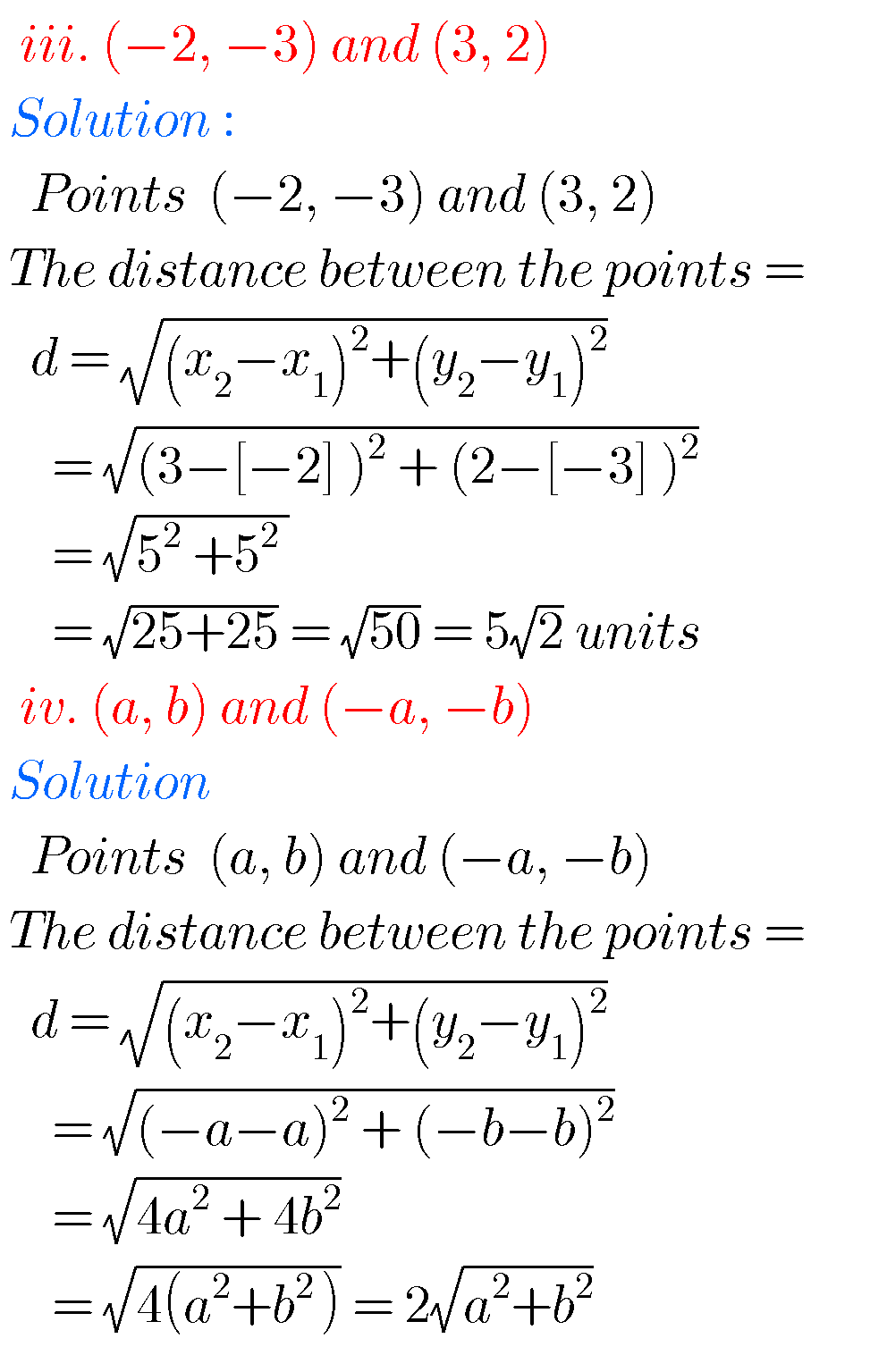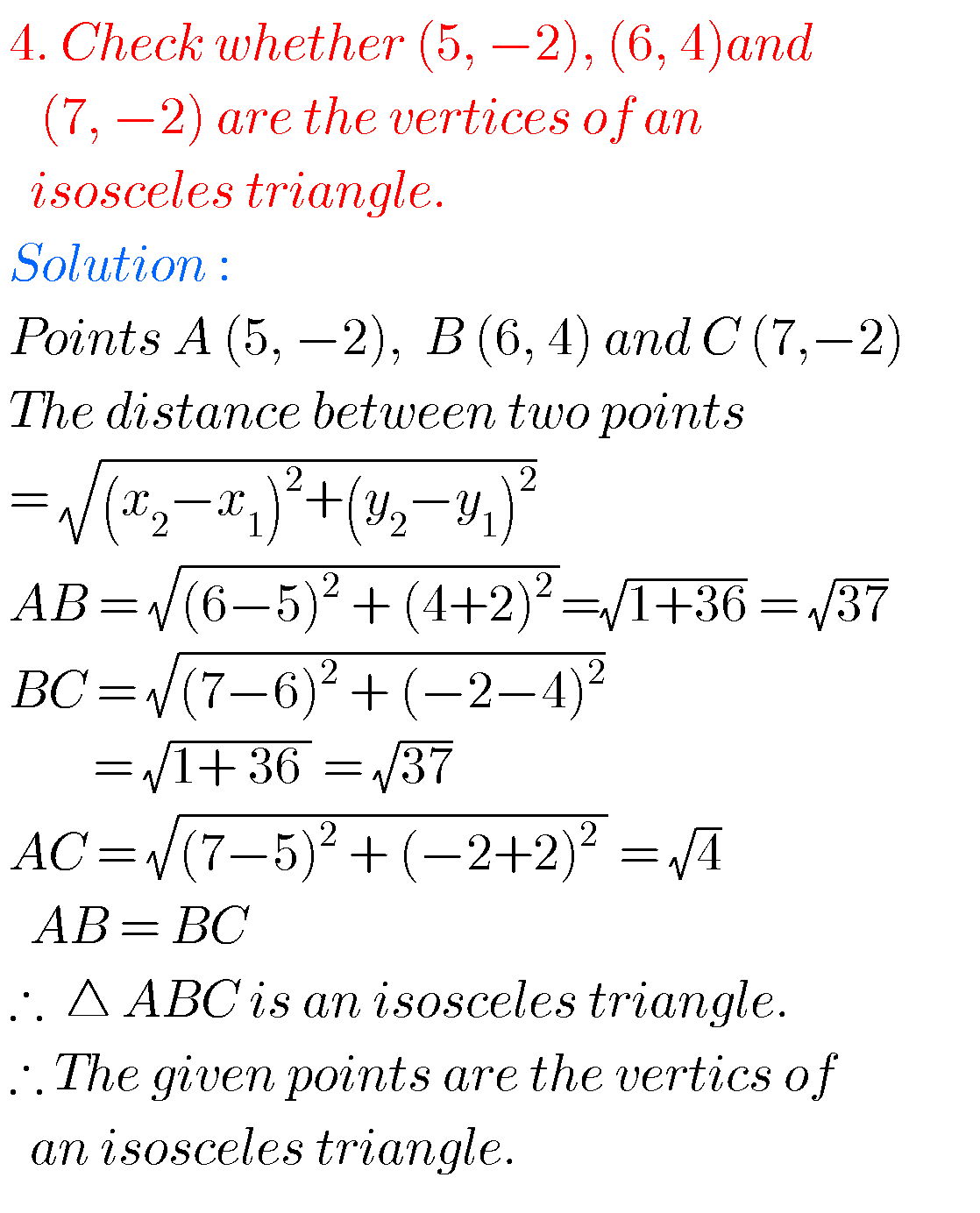F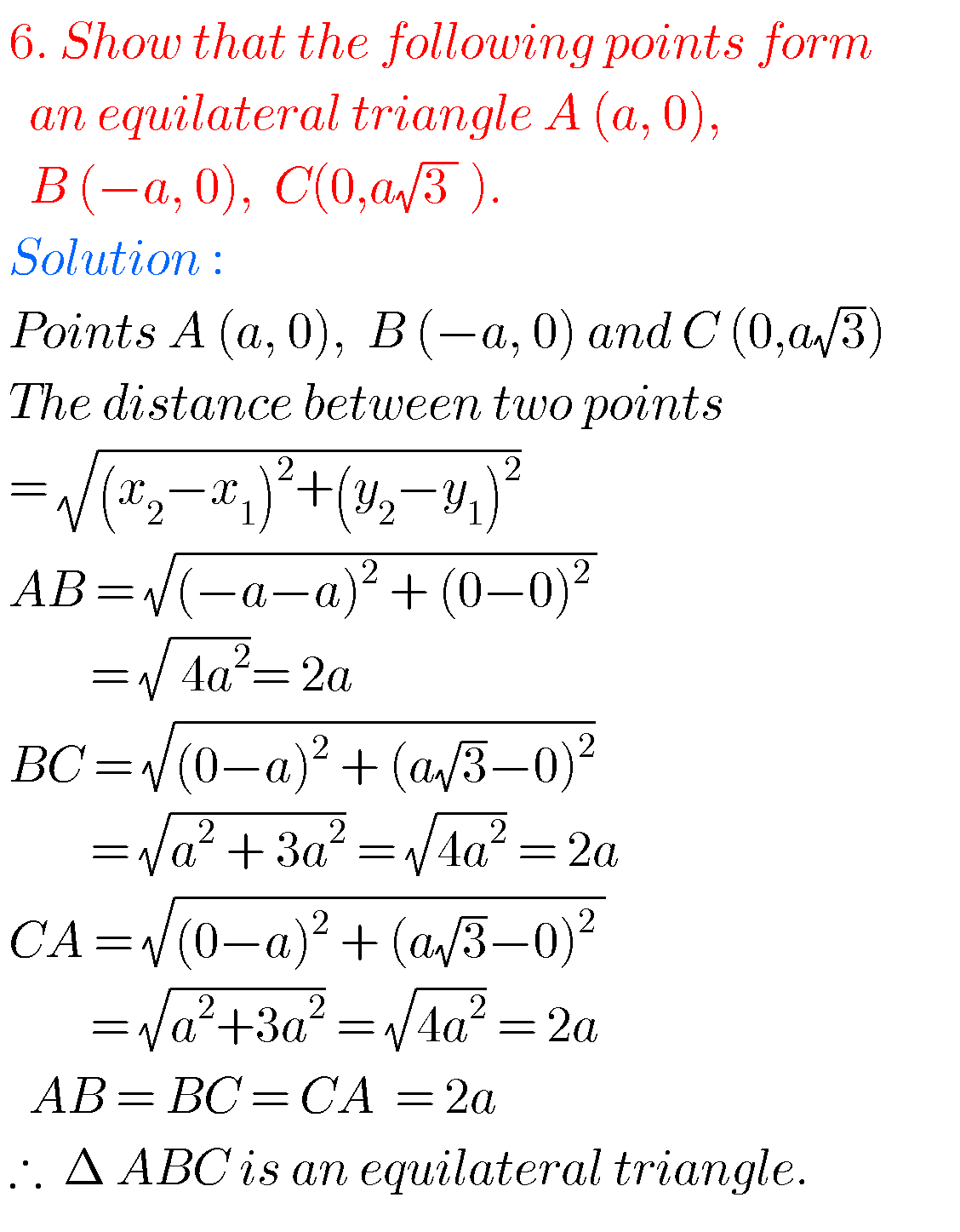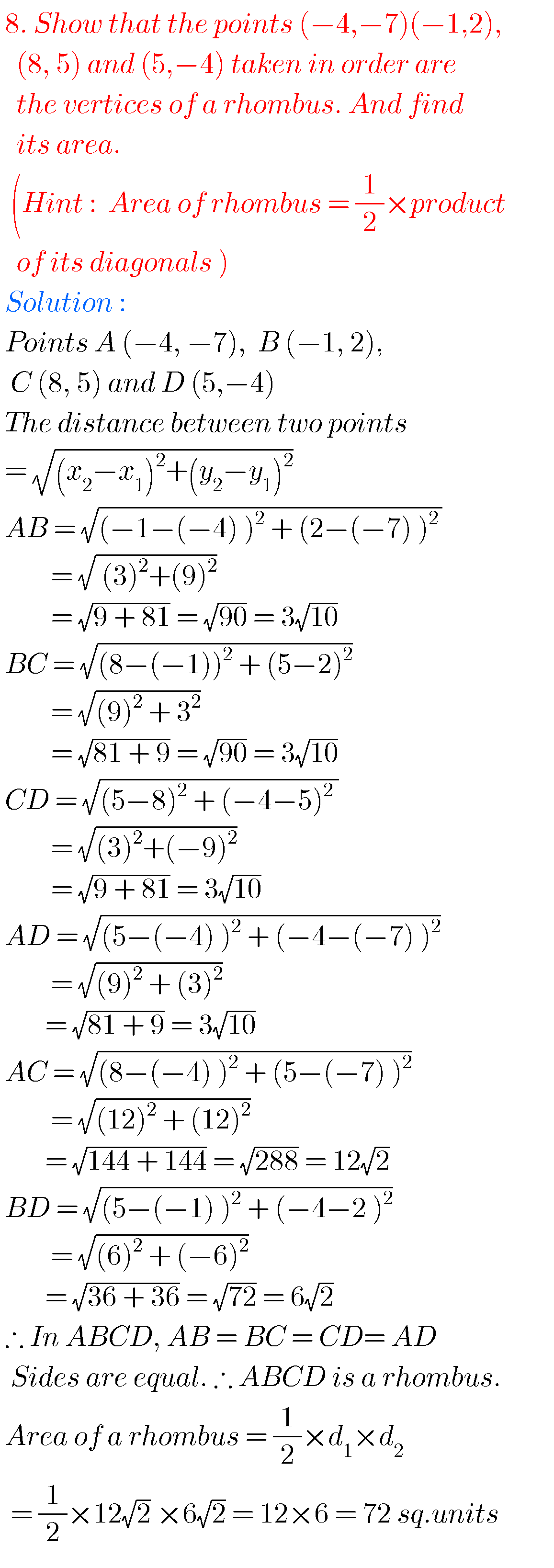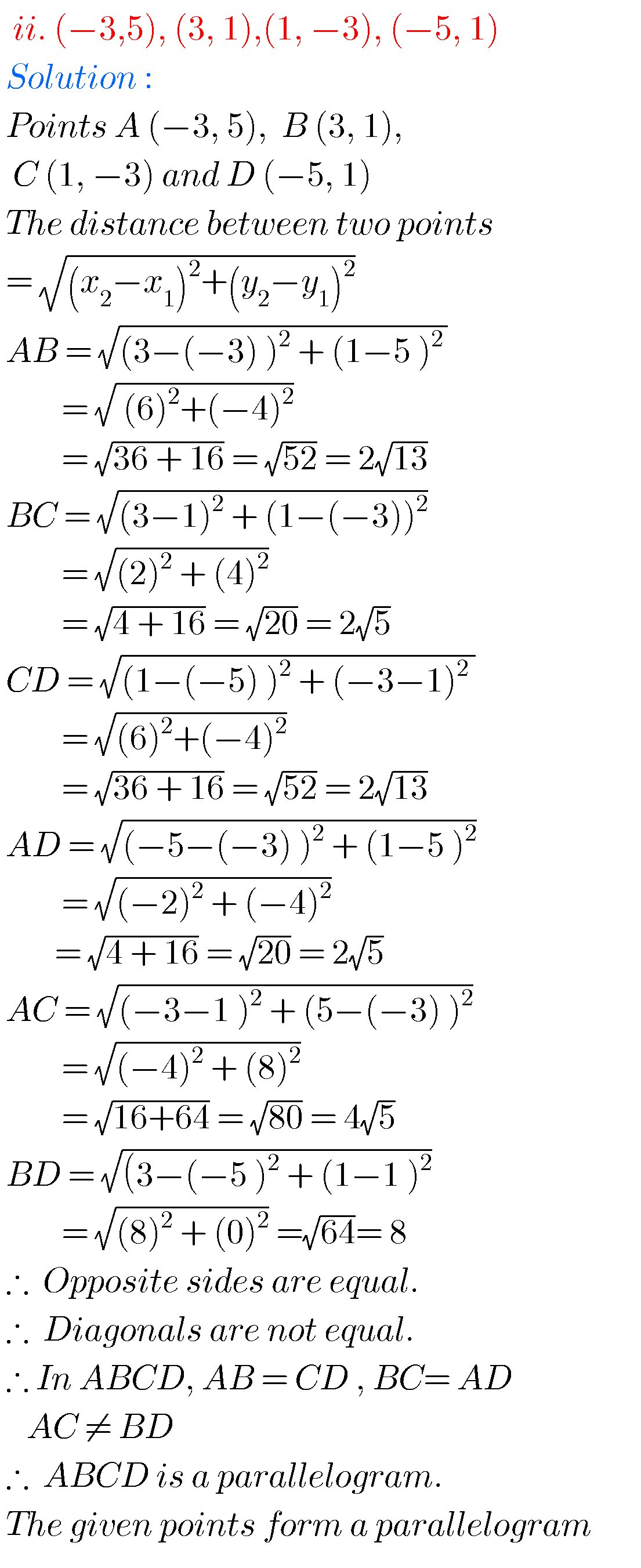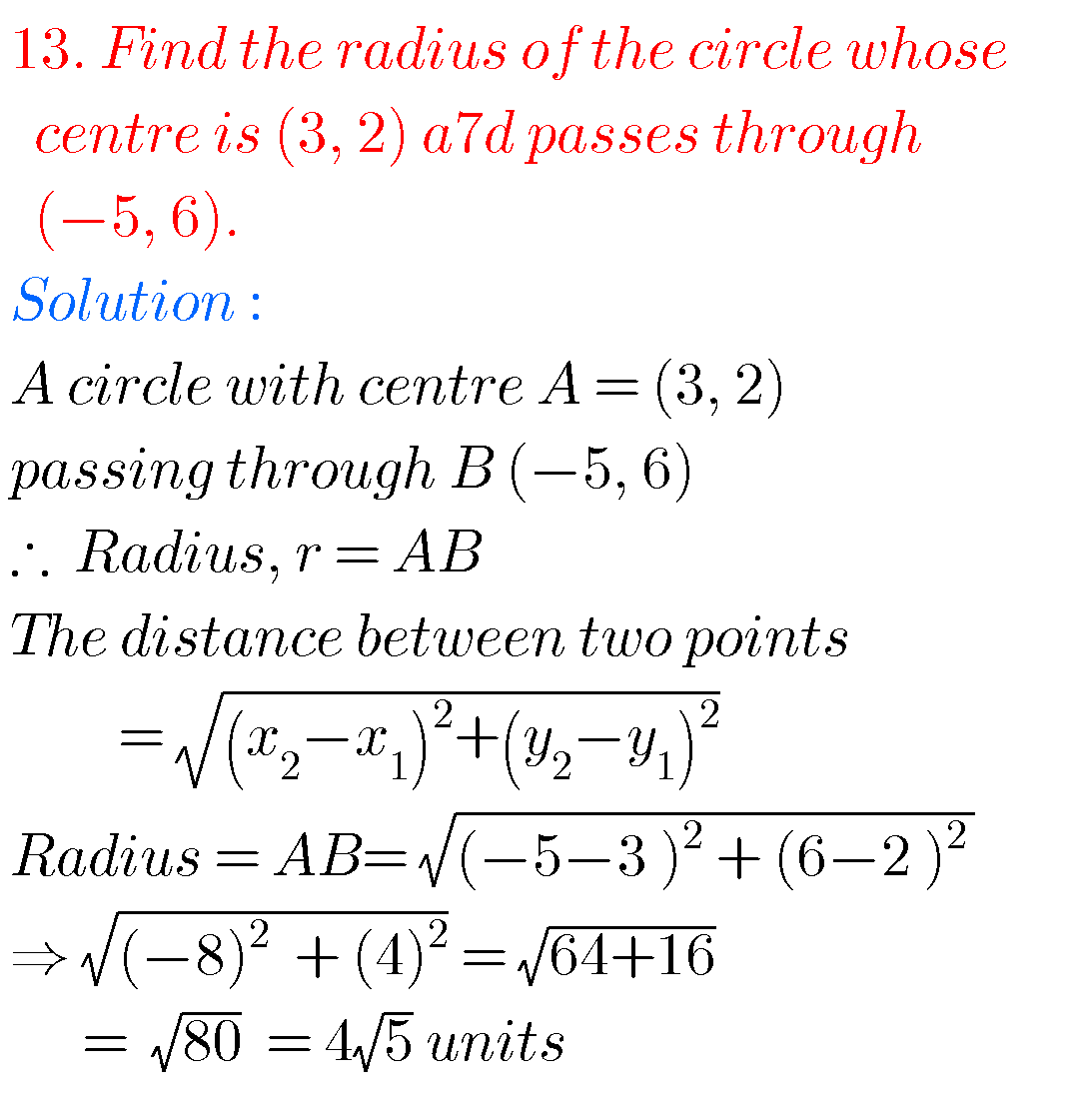## Maths solutions for Coordinate Geometry class 10 SSC

Chapter 7  ssc  solutions

Exercise 7.2 class 10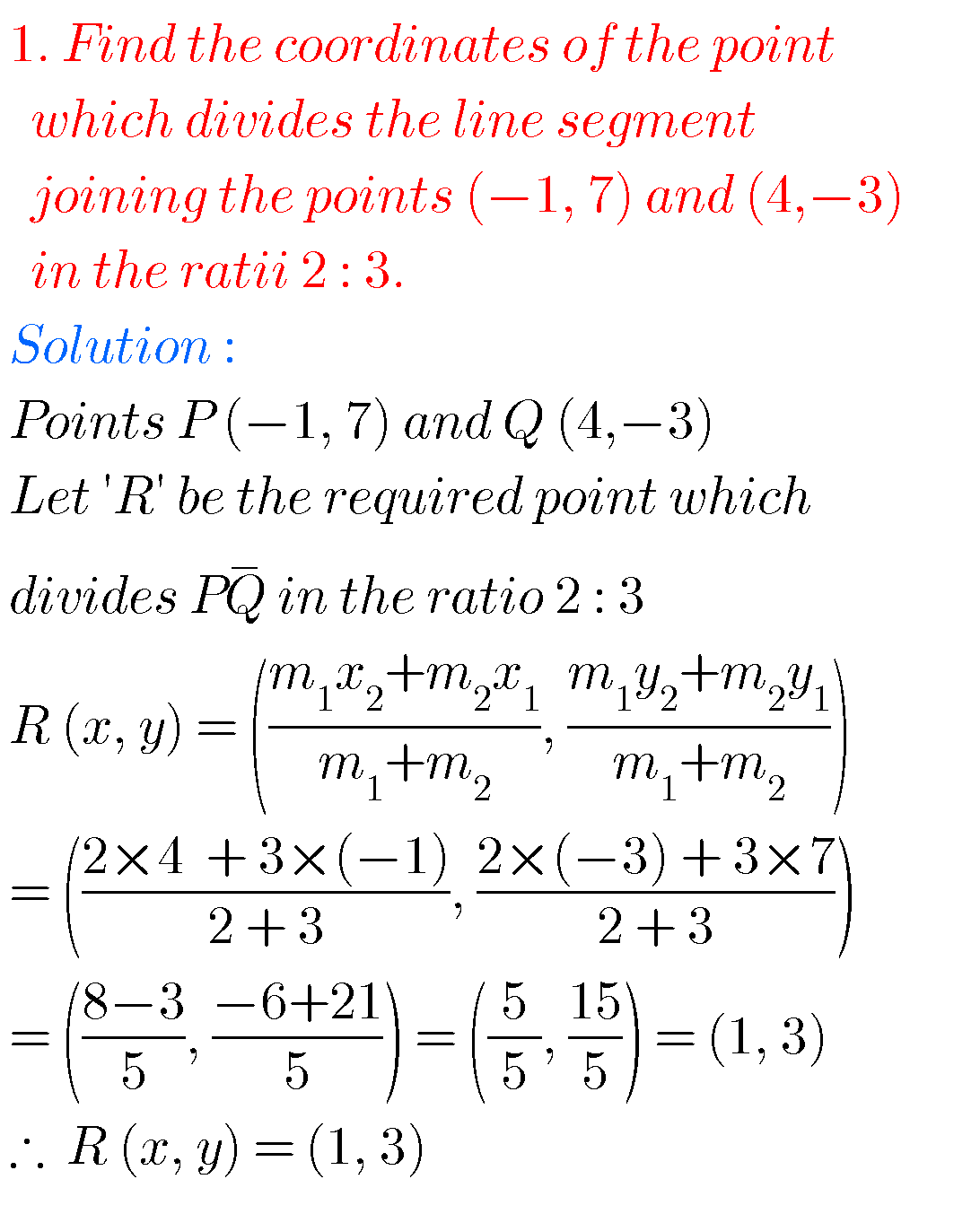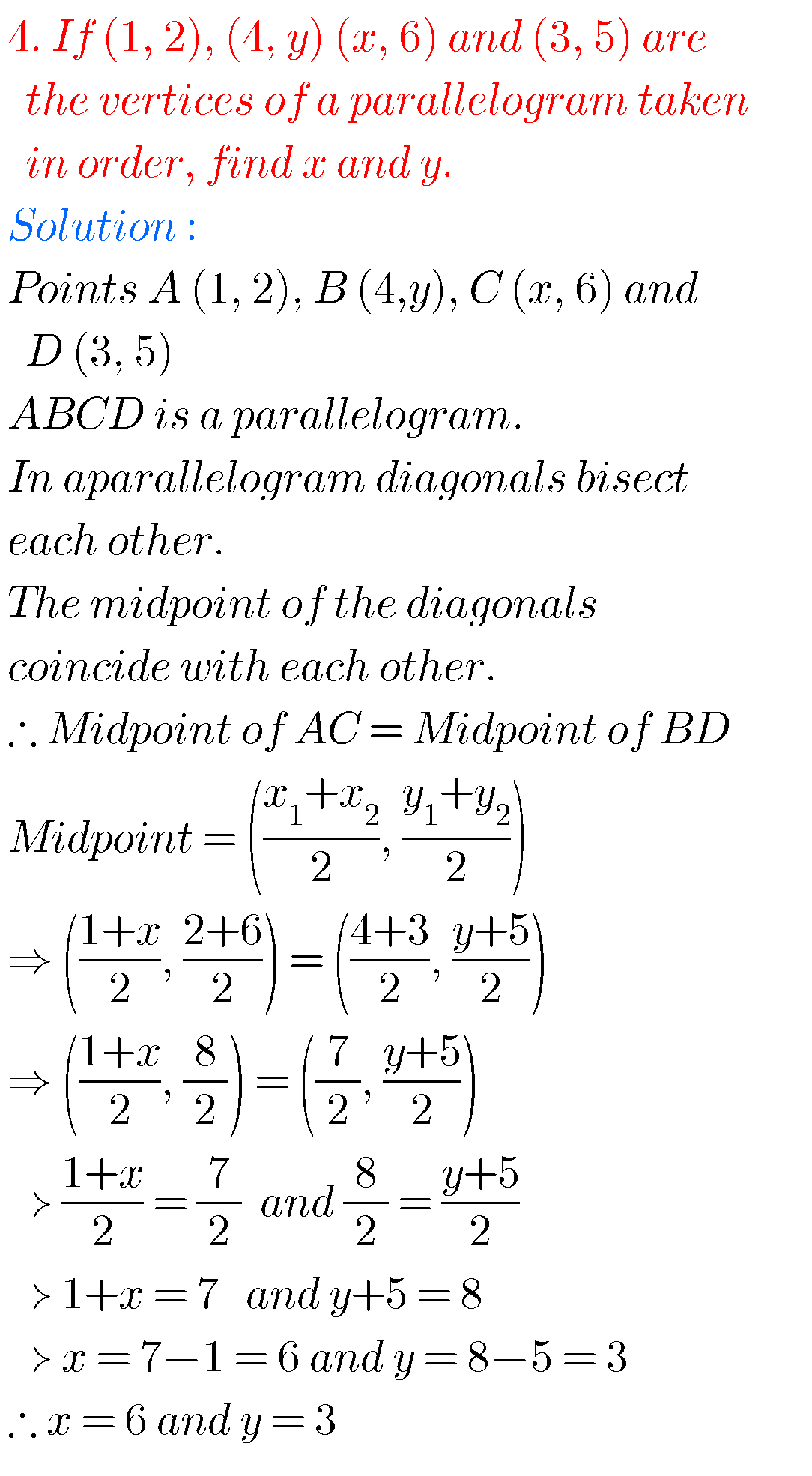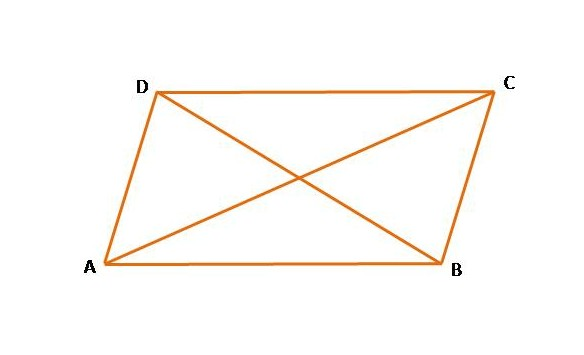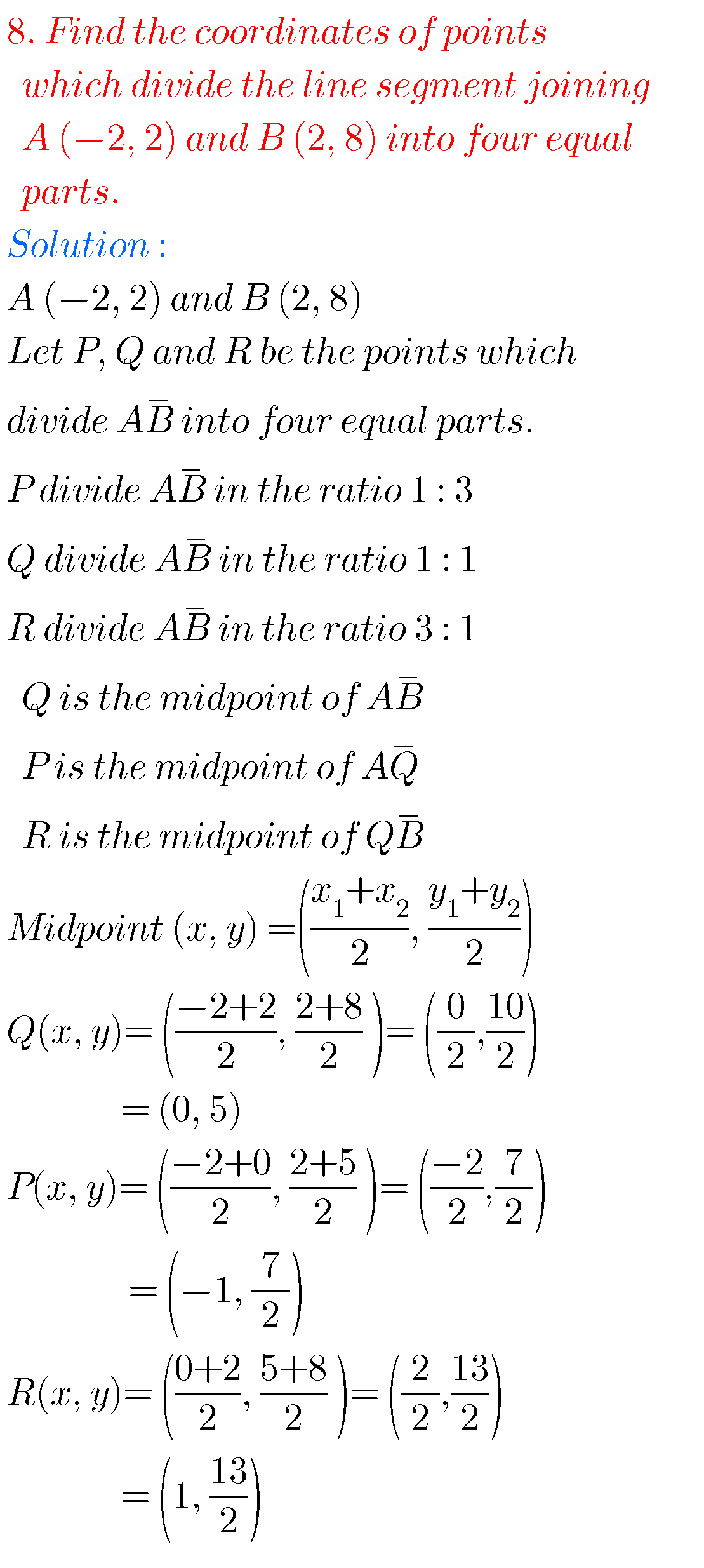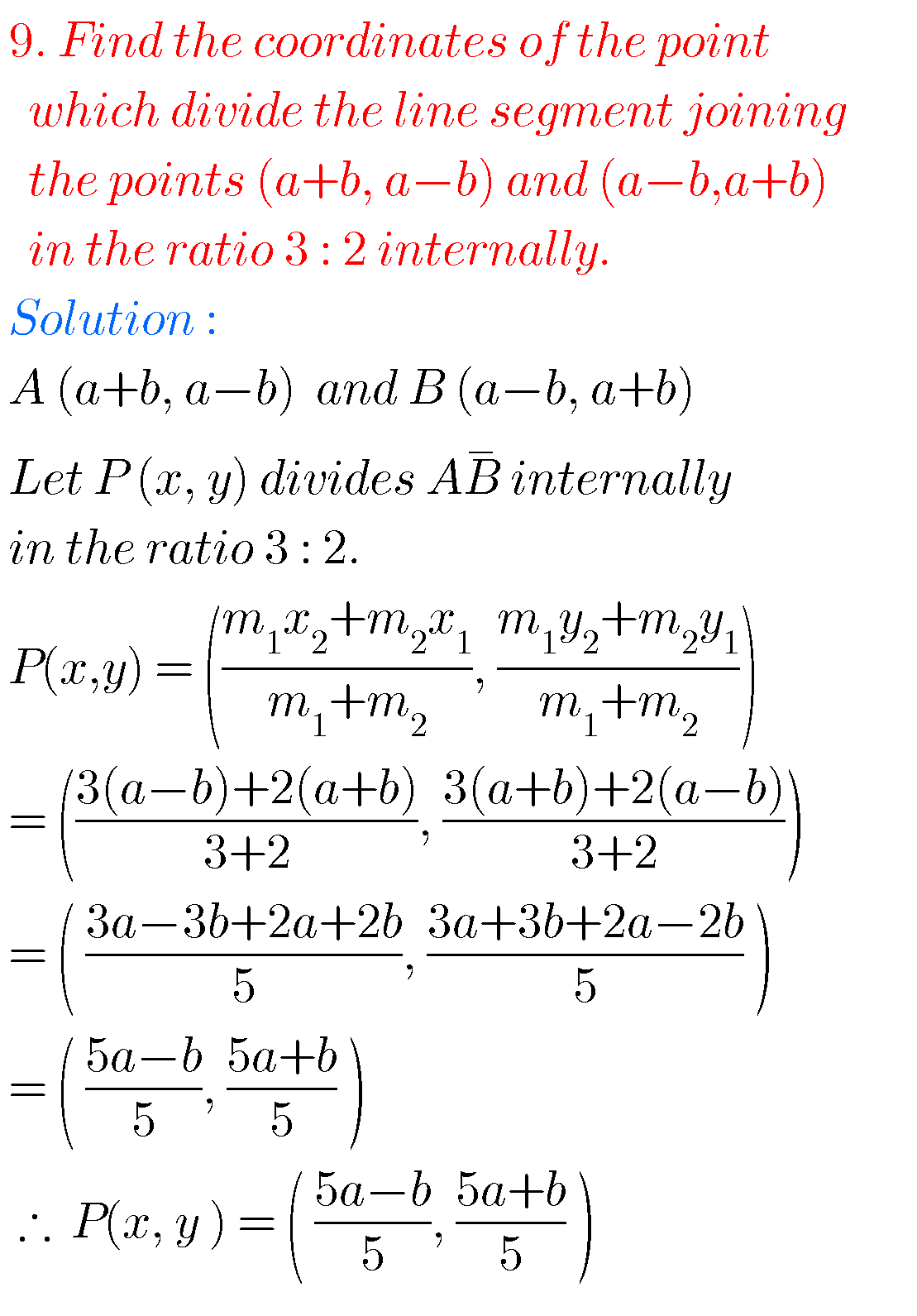## Solutions for Coordinate Geometry SSC class 10

Exercise 7.3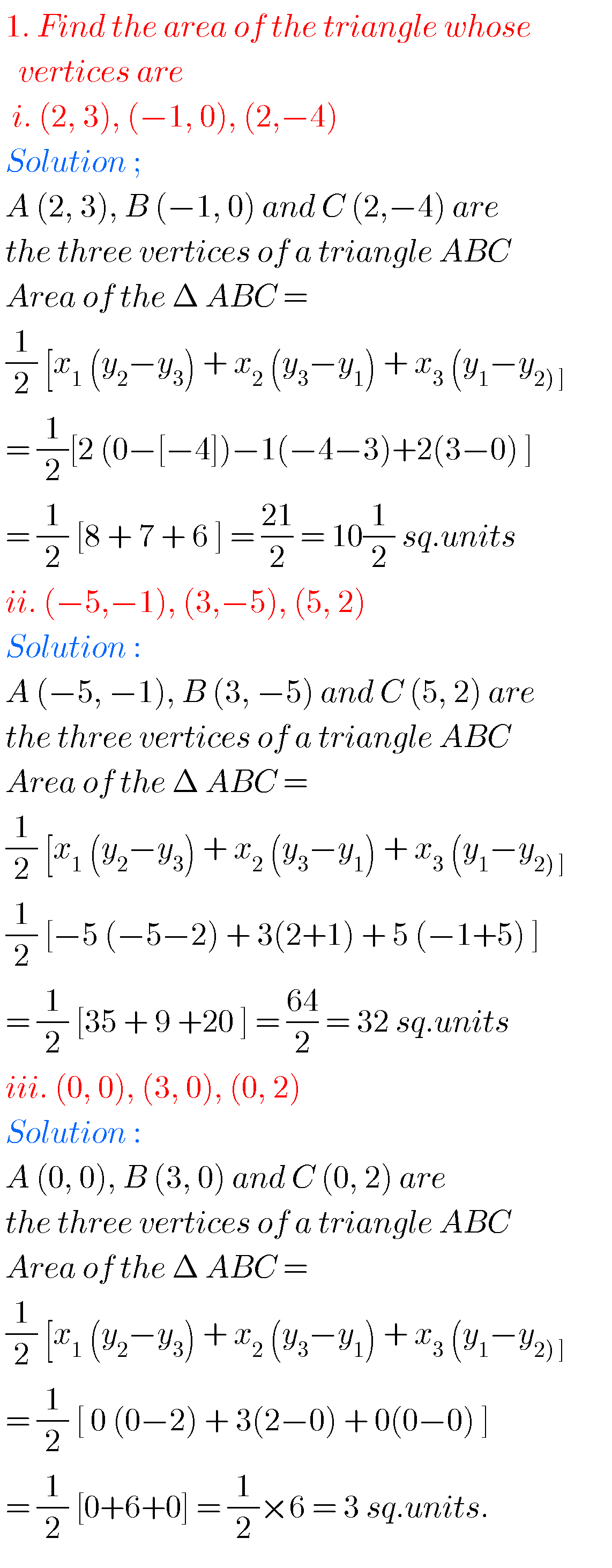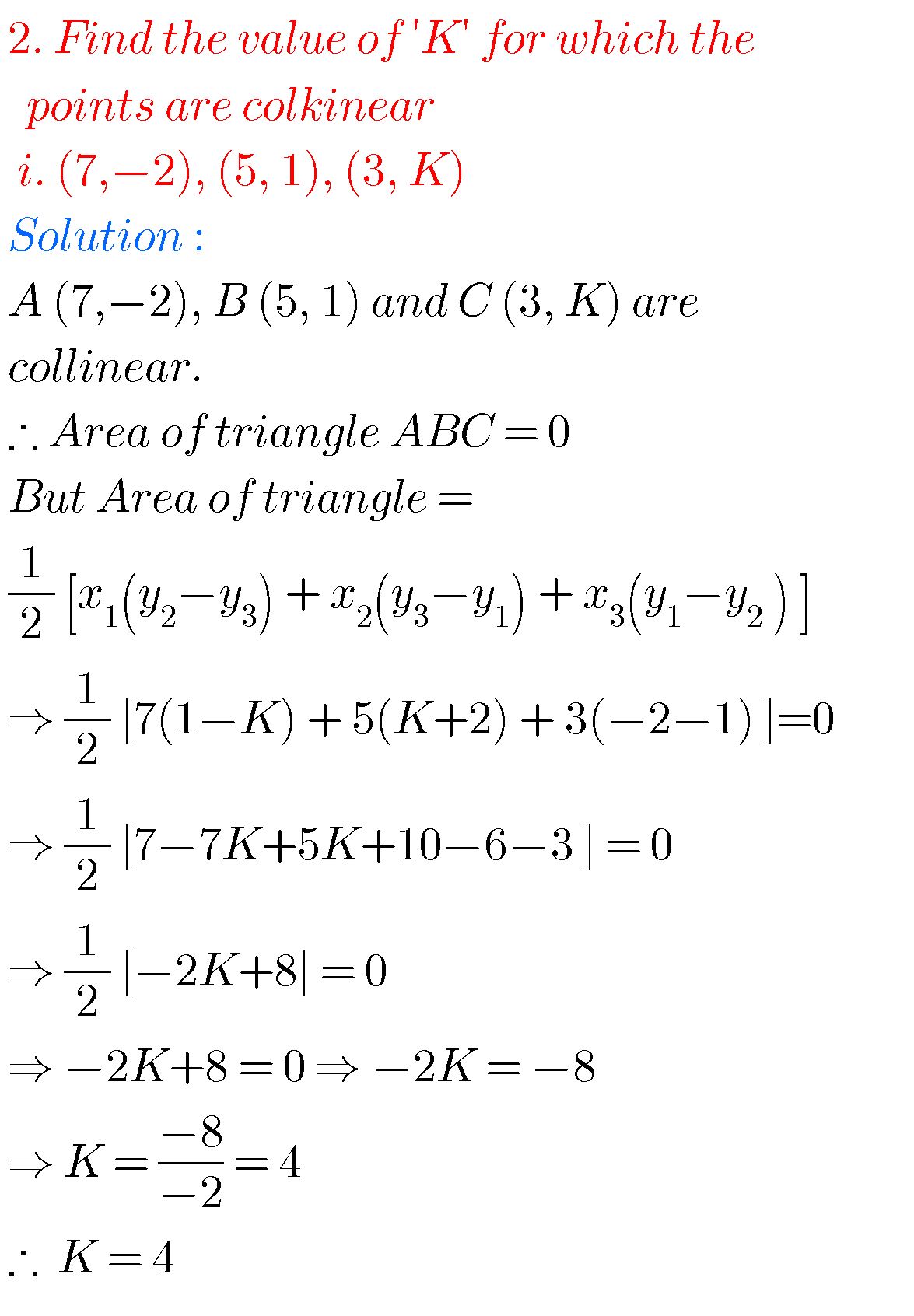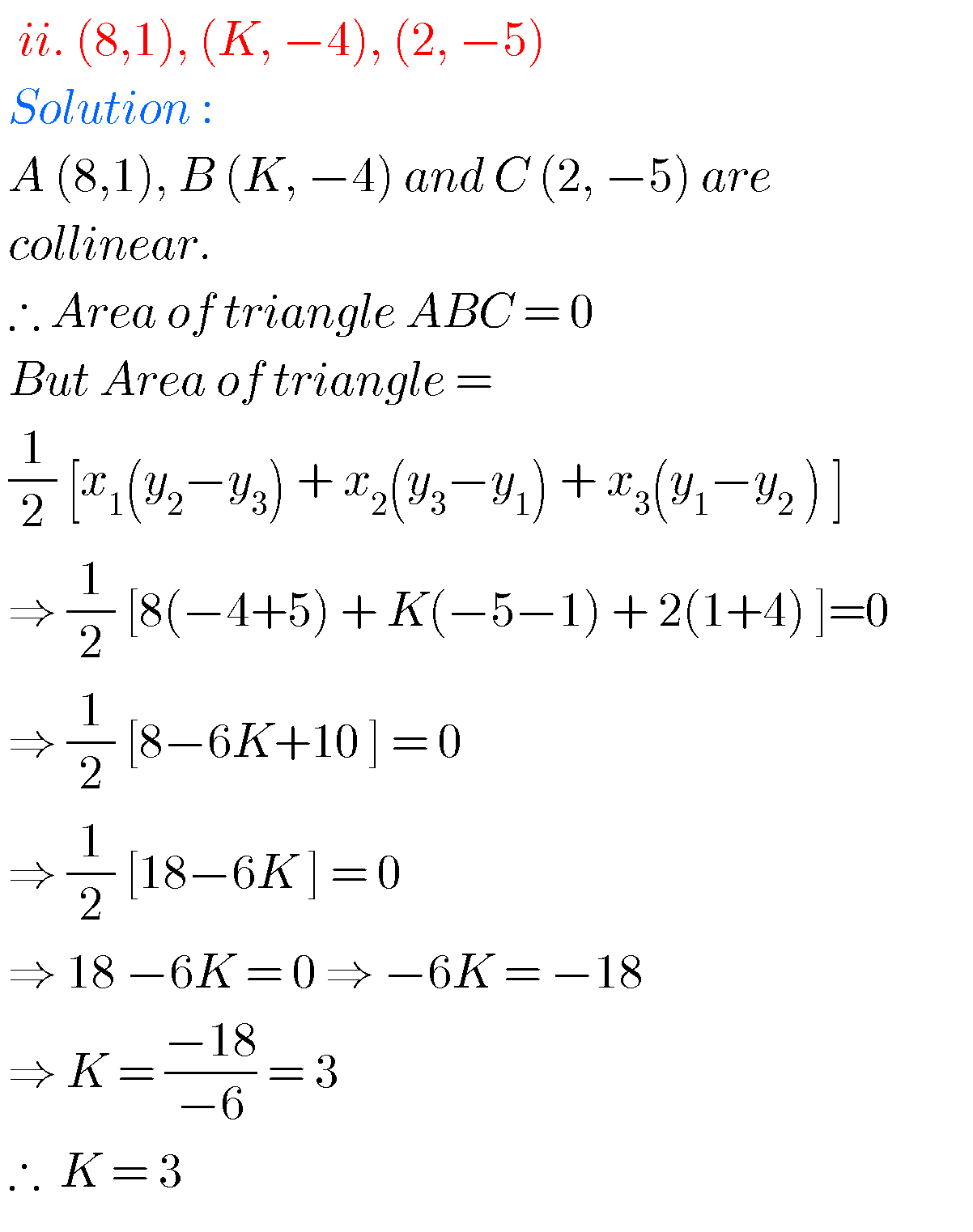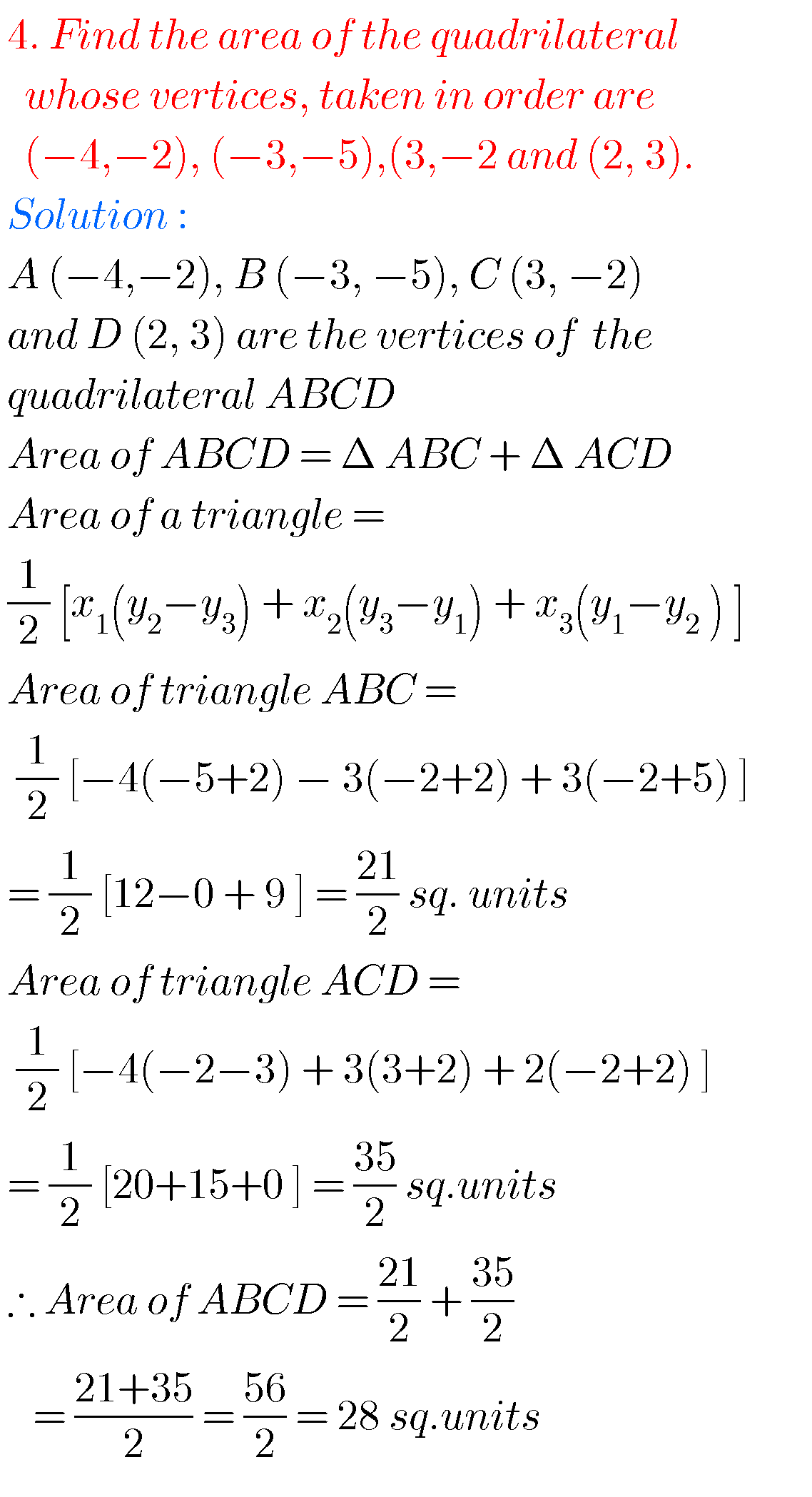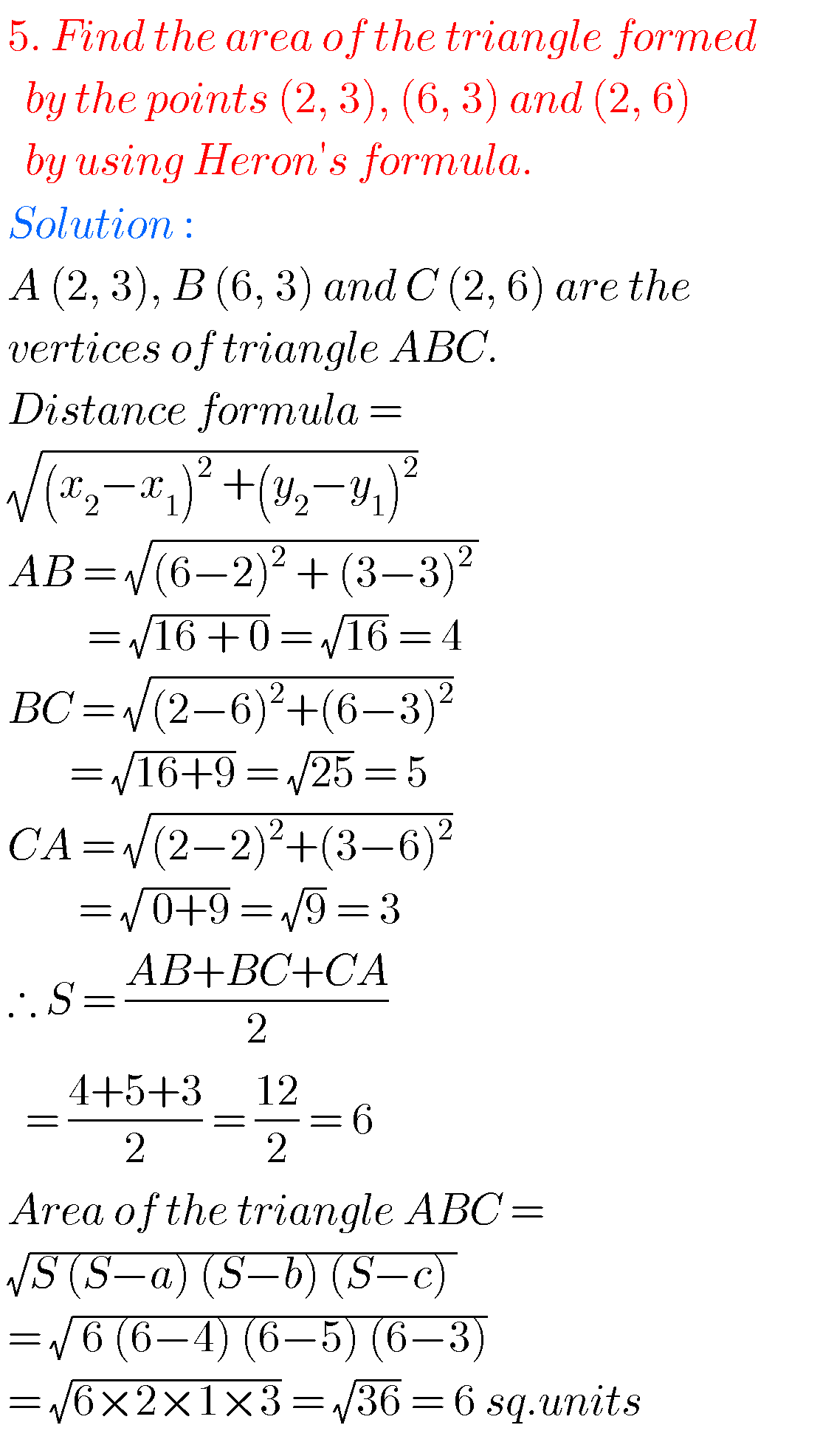### 10th class maths solutions for Coordinate Geometry

Exercise 7.4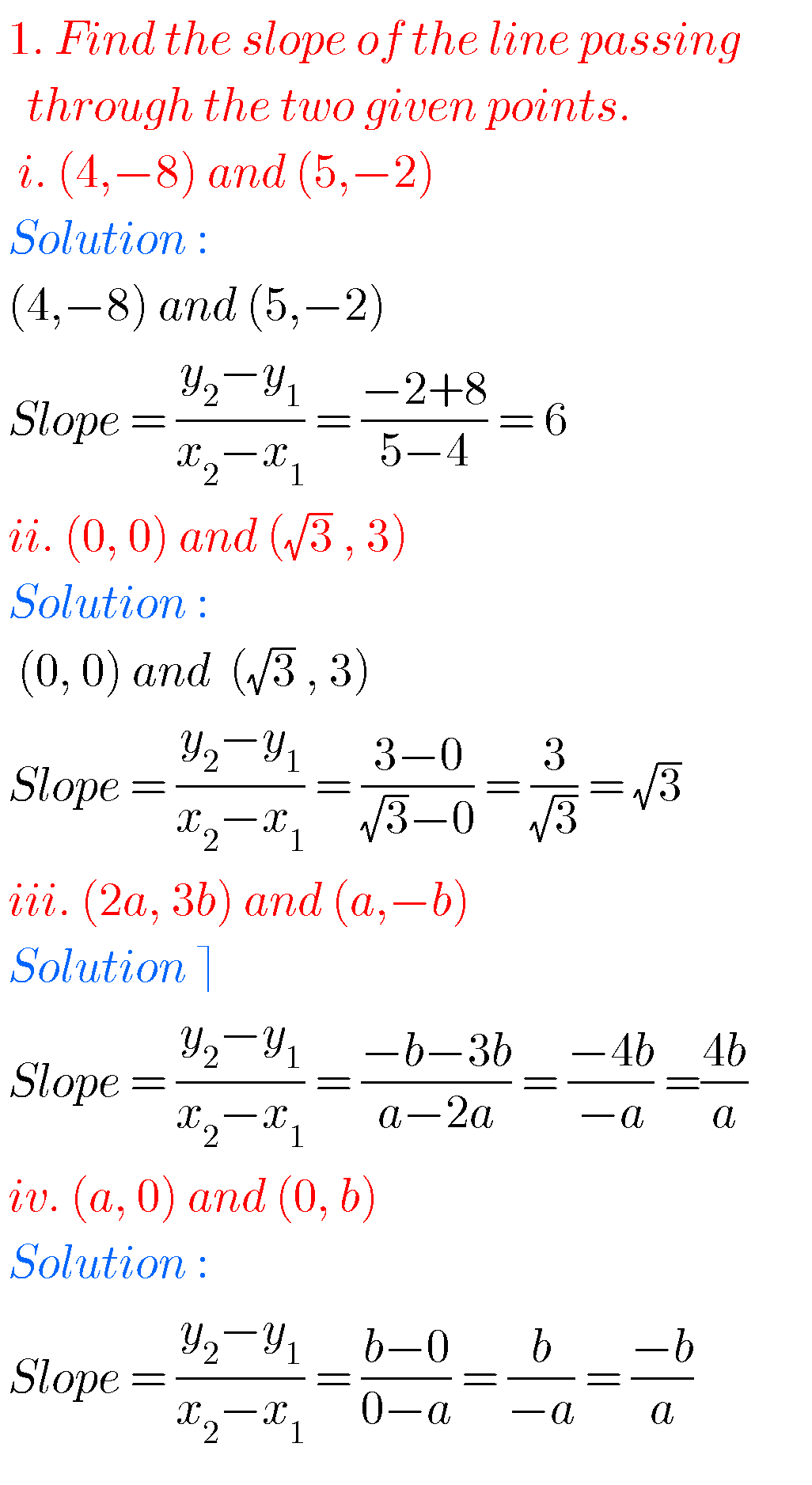Optional Exercise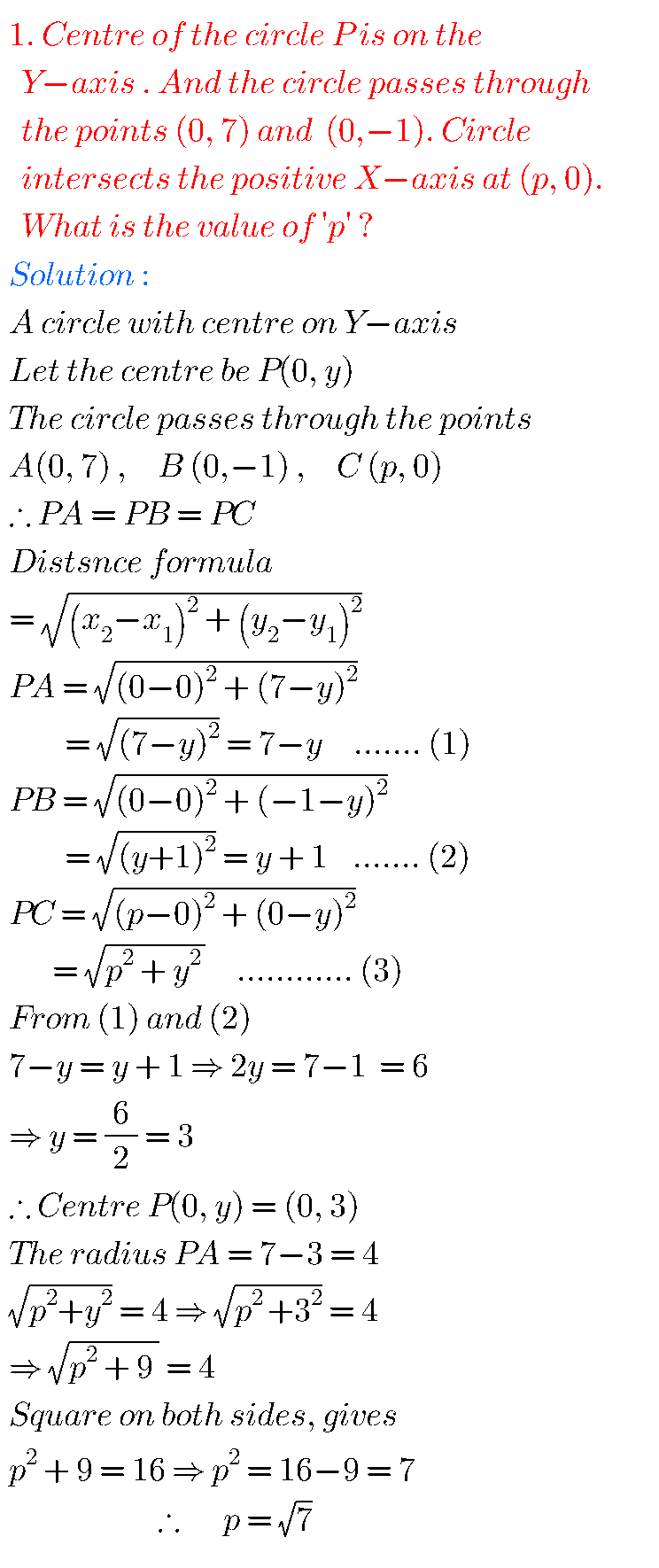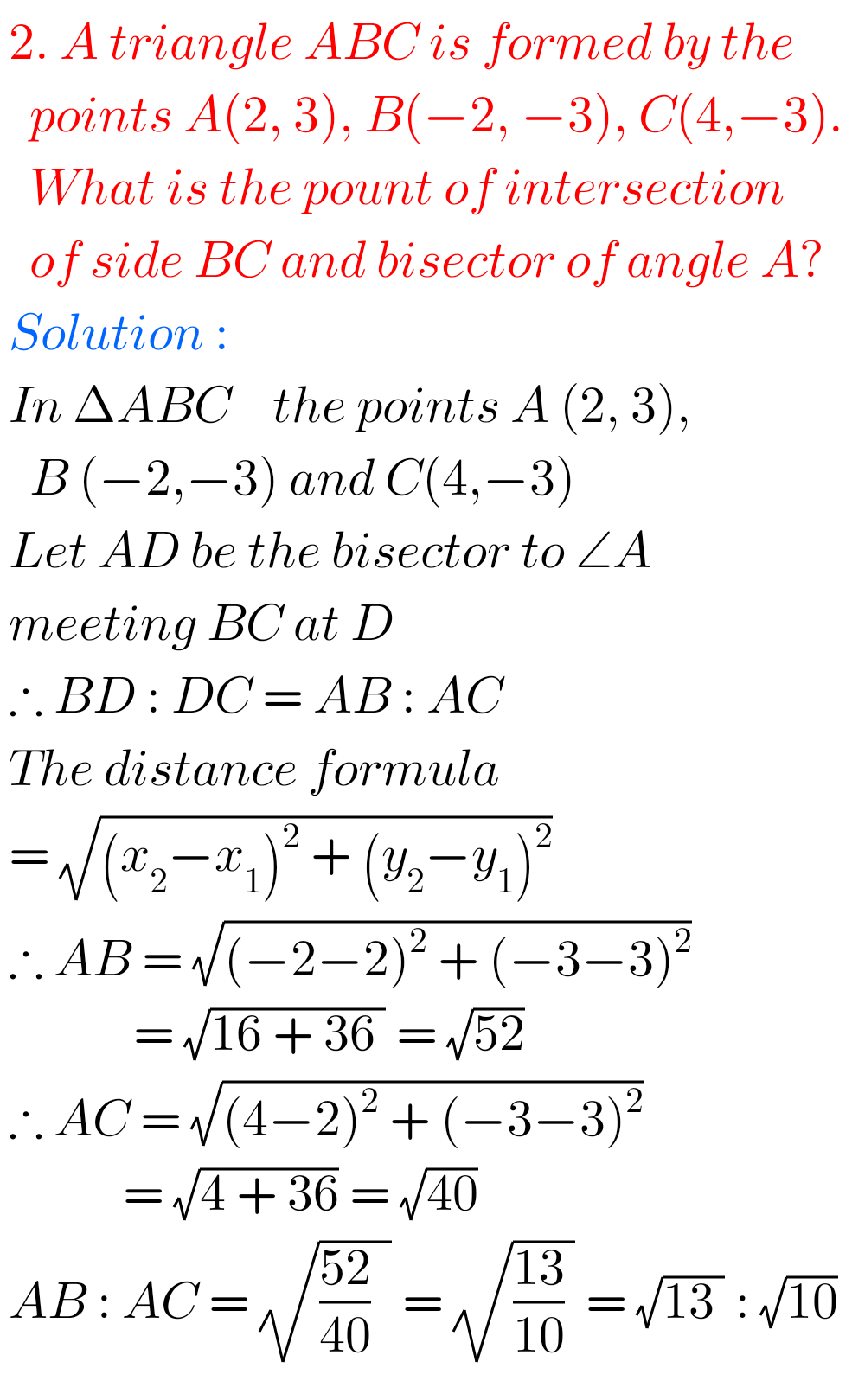M

Note : Observe the solutions and try them in your own method.

You can also see the solutions for inter

Inter maths solutions for 1A for exam purpose

3. Matrices

You can also see the solutions for 1b

1. Locus

The straight line sa

Straight lines la

You can see solutions for Inter Maths IIB

1. Circle

3. Parabola

4. Ellipse

You can also see solutions for Inter Maths IIA

For examination purpose you can see

Complex numbers

De Moivre’ s Theorem

You can see the solutions for text book Maths 1A

Functions

Exercise 1(a)

Exercise 1(b)

Exercise 1(c)

Mathematical Induction

Exercise 2(a)

Matrices

Exercise 3(a)

Exercise 3(b)

Exercise 3(c)

Exercise 3(d)

Exercise 3(e)

Exercise 3(f)

Exercise 3(g)

Trigonometric Ratios up to Transformations

Exercise 6(a)

Exercise 6(b)

Exercise 6(c)

Exercise 6(d)

Exercise 6(e)

Exercise 6(f)

Trigonometric Equations

Exercise 7(a)

Inverse Trigonometric Equations

Exercise 8(a)

Hyperbolic Functions

Exercise 9(a)

Properties of Triangles

Exercise 10(a)

Exercise 10(b)

1.•2.